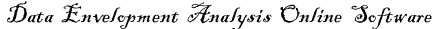# CCR model

In the DEA methodology, formerly developed by Charnes, Cooper and Rhodes (1978) (CCR), efficiency is defined as a weighted sum of outputs to a weighted sum of inputs, where the weights structure is calculated by means of mathematical programming and constant returns to scale (CRS) are assumed.

input orientedCharnes, A., Cooper, W.W., and Rhodes, E., 1978, "Measuring The Efficiency of Decision Making Units," European Journal of Operational Research, 2, 429-444.